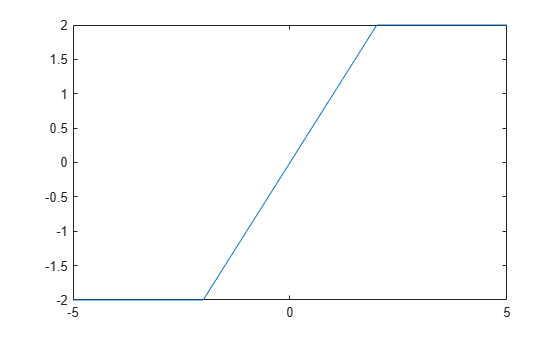piecewise

Conditionally defined expression or function

Description

example

pw = piecewise(cond1,val1,cond2,val2,...) returns the piecewise expression or function pw whose value is val1 when condition cond1 is true, is val2 when cond2 is true, and so on. If no condition is true, the value of pw is NaN.

example

pw = piecewise(cond1,val1,cond2,val2,...,otherwiseVal) returns the piecewise expression or function pw that has the value otherwiseVal if no condition is true.

Examples

collapse all

Define the following piecewise expression by using piecewise.

$\mathit{y}=\left\{\begin{array}{ll}-1& \mathit{x}<0\\ 1& \mathit{x}>0\end{array}$

syms x
y = piecewise(x < 0,-1,x > 0,1)
y =

Evaluate y at -2, 0, and 2 by using subs to substitute for x. Because y is undefined at x = 0, the value is NaN.

subs(y,x,[-2 0 2])
ans = $\left(\begin{array}{ccc}-1& \mathrm{NaN}& 1\end{array}\right)$

Define the following function symbolically.

$\mathit{y}\left(\mathit{x}\right)=\left\{\begin{array}{ll}-1& \mathit{x}<0\\ 1& \mathit{x}>0\end{array}$

syms y(x)
y(x) = piecewise(x < 0,-1,x > 0,1)
y(x) =

Because y(x) is a symbolic function, you can directly evaluate it for values of x. Evaluate y(x) at -2, 0, and 2. Because y(x) is undefined at x = 0, the value is NaN. For details, see Create Symbolic Functions.

y([-2 0 2])
ans = $\left(\begin{array}{ccc}-1& \mathrm{NaN}& 1\end{array}\right)$

Set the value of a piecewise function when no condition is true (called otherwise value) by specifying an additional input argument. If an additional argument is not specified, the default otherwise value of the function is NaN.

Define the piecewise function

$y=\left\{\begin{array}{cc}-2& x<-2\\ 0& -2

syms y(x)
y(x) = piecewise(x < -2,-2,(-2 < x) & (x < 0),0,1)
y(x) =

Evaluate y(x) between -3 and 1 by generating values of x using linspace. At -2 and 0, y(x) evaluates to 1 because the other conditions are not true.

xvalues = linspace(-3,1,5)
xvalues = 1×5

-3    -2    -1     0     1

yvalues = y(xvalues)
yvalues = $\left(\begin{array}{ccccc}-2& 1& 0& 1& 1\end{array}\right)$

Plot the following piecewise expression by using fplot.

$y=\left\{\begin{array}{cc}-2& x<-2\\ x& -22\end{array}.$

syms x
y = piecewise(x < -2,-2,-2 < x < 2,x,x > 2,2);
fplot(y)On creation, a piecewise expression applies existing assumptions. Apply assumptions set after creating the piecewise expression by using simplify on the expression.

Assume x > 0. Then define a piecewise expression with the same condition x > 0. piecewise automatically applies the assumption to simplify the condition.

syms x
assume(x > 0)
pw = piecewise(x < 0,-1,x > 0,1)
pw = $1$

Clear the assumption on x for further computations.

assume(x,'clear')

Create a piecewise expression pw with the condition x > 0. Then set the assumption that x > 0. Apply the assumption to pw by using simplify.

pw = piecewise(x < 0,-1,x > 0,1);
assume(x > 0)
pw = simplify(pw)
pw = $1$

Clear the assumption on x for further computations.

assume(x,'clear')

Differentiate, integrate, and find limits of a piecewise expression by using diff, int, and limit respectively.

Differentiate the following piecewise expression by using diff.

$\mathit{y}=\left\{\begin{array}{ll}1/\mathit{x}& \mathit{x}<-1\\ \mathrm{sin}\left(\mathit{x}\right)/\mathit{x}& \mathit{x}\ge -1\end{array}$

syms x
y = piecewise(x < -1,1/x,x >= -1,sin(x)/x);
diffy = diff(y,x)
diffy =

Integrate y by using int.

inty = int(y,x)
inty =

Find the limits of y at 0 by using limit.

limit(y,x,0)
ans = $1$

Find the right- and left-sided limits of y at -1. For details, see limit.

limit(y,x,-1,'right')
ans = $\mathrm{sin}\left(1\right)$
limit(y,x,-1,'left')
ans = $-1$

Add, subtract, divide, and multiply two piecewise expressions. The resulting piecewise expression is only defined where the initial piecewise expressions are defined.

syms x
pw1 = piecewise(x < -1,-1,x >= -1,1);
pw2 = piecewise(x < 0,-2,x >= 0,2);

sub = pw1-pw2
sub =

mul = pw1*pw2
mul =

div = pw1/pw2
div =

Modify a piecewise expression by replacing part of the expression using subs. Extend a piecewise expression by specifying the expression as the otherwise value of a new piecewise expression. This action combines the two piecewise expressions. piecewise does not check for overlapping or conflicting conditions. Instead, like an if-else ladder, piecewise returns the value for the first true condition.

Change the condition x < 2 in a piecewise expression to x < 0 by using subs.

syms x
pw = piecewise(x < 2,-1,x > 0,1);
pw = subs(pw,x < 2,x < 0)
pw =

Add the condition x > 5 with the value 1/x to pw by creating a new piecewise expression with pw as the otherwise value.

pw = piecewise(x > 5,1/x,pw)
pw =

Input Arguments

collapse all

Condition, specified as a symbolic condition or variable. A symbolic variable represents an unknown condition.

Example: x > 2

Value when condition is satisfied, specified as a number, vector, matrix, or multidimensional array, or as a symbolic number, variable, vector, matrix, multidimensional array, function, or expression.

Value if no conditions are true, specified as a number, vector, matrix, or multidimensional array, or as a symbolic number, variable, vector, matrix, multidimensional array, function, or expression. If otherwiseVal is not specified, its value is NaN.

Output Arguments

collapse all

Piecewise expression or function, returned as a symbolic expression or function. The value of pw is the value val of the first condition cond that is true. To find the value of pw, use subs to substitute for variables in pw.

Tips

• piecewise does not check for overlapping or conflicting conditions. A piecewise expression returns the value of the first true condition and disregards any following true expressions. Thus, piecewise mimics an if-else ladder.